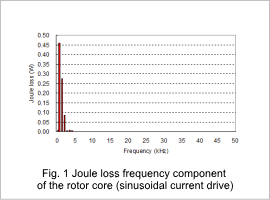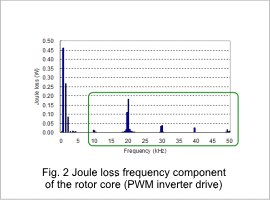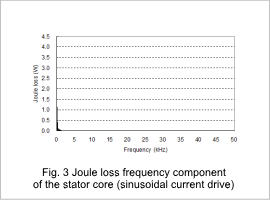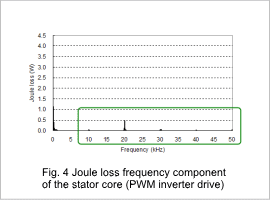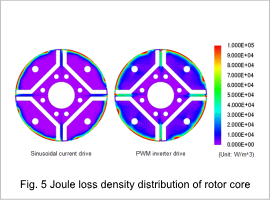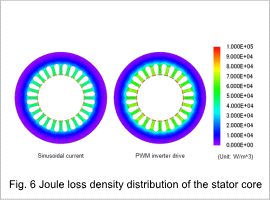## [JAC059] Iron Loss Analysis of an IPM Motor Accounting for PWN –Direct Link-

Remember me

*It is different from the service for JMAG WEB MEMBER (free member). Please be careful.
About authentication ID for JMAG website

### Overview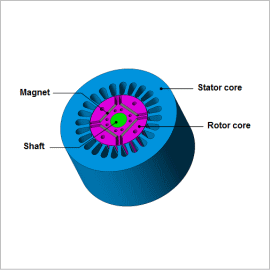Vector controls using a PWM (Pulse Width Modulation) control are commonly included in the drive circuits of high efficiency motors. A PWM control makes it possible to adjust the phase or amplitude of a current according to load and rotation speed, so they can achieve high efficiency in a wide operation range. The control frequency of a PWM is called a carrier frequency. Carrier frequencies are often used up to almost 20 kHz. To form the current waveform supplied by the PWM control, the carrier harmonic current is superimposed on the basic wave current. This carrier harmonic current applies a high-frequency magnetic field to each part of the motor. As a result, core iron loss and magnet eddy current loss are generated.
The total amount of these losses is not a dominant factor, but they can be a hindrance when trying to raise efficiency, so they need to be eliminated in the design process. In order to study these problems, both the motor’s electromagnetic behavior and what kinds of controls the drive circuit performs have to be investigated.
In order to run an advance study of these phenomena in CAE, a high fidelity motor model and inverter model need to be coupled. There are three ways of accomplishing this: Directly linking with a circuit/control simulator, entering a current waveform obtained by using a JMAG-RT motor model and a circuit/control simulator, and entering actual current measurements.
In this analysis, the iron losses of the IPM motor that accounts for the carrier harmonic are obtained by directly linking to a circuit/control simulator.

### Iron LossesComparisons of the iron losses, hysteresis losses, and joule losses when the IPM motor is driven by a PWM inverter and a sinusoidal current are shown in table 1.
The iron losses in both the rotor core and the stator core increase when the IPM motor is run using a PWM inverter. The joule losses increase the most severely, increasing 70% in the rotor core, and 40% in the stator core.

### Joule Loss

The frequency components of the joule losses are indicated in figures 1 through 4. The joule loss density distribution is indicated in figures 5 and 6.
The fundamental frequency and harmonic component have almost the same amount of iron losses in both the rotor core and the stator core if a sinusoidal current or a PWM inverter is used with a fundamental frequency of 360 Hz for the rotor core and 60 Hz stator core. However, losses are only generated when the PWM inverter is used with a PWM fundamental frequency of 10 kHz and its harmonic component. (These values are indicated inside the green square in fig. 1.)
The increased joule losses when the motor is run using a PWM inverter are caused by the carrier harmonic. The effects of a carrier harmonic need to be accounted for to analyze the characteristics of an IPM motor in detail.